# CS101 - Homework 4

Due: 2018-03-14 11:59p

#### Objectives

• Gain more practice with recursion and the turtle
• Learn about `random`

### [10 points] Prelab

Before coming to lab on Thursday / Friday, read this entire homework write-up and complete the prelab.

### [5 points] Incorporate random

Once you have the tree drawing correctly, and you have tested a couple of generations, we are going to start making it more tree-like.

We will start by adding a random element to the drawing. We will do this with the help of the `random` library. The `random` library provides us with a random number generator, which will allow us to generate seemingly random numbers the way we would roll a die. In truth, there is an algorithmic process that governs the sequence of numbers, so it is not truly random, but it is close enough for us right now. The main feature we are using from the library is a function that will return random integers within some range that we can specify.

• Step 1: Import the random library.
``````import random
``````
• Step 2: Call the random integer generating function. The function name is `randint`. It takes two arguments that define the range of the random numbers that we want. The first gives the start of the range, and the second is the end. If we call the function `randint(3,7)`, the function will return a number that is either 3,4,5,6, or 7. Each time you call the function, you will get any one number from that set. Because the function is in a library, we need to preface it with the name of the library. So, if I wanted a number between 5 and 9 inclusive, I would write something like this:
``````random.randint(5,9)
``````

Type the above two lines in on the Python console in Thonny. Run the function a couple of times until you feel comfortable with what it is doing.

Now that you can generate random numbers, use it to randomize the tree. There are two main things we can randomize: the length of a particular branch, and the angle with respect to the trunk.

Some ideas:

To randomize the trunk length, you could add a random value, say between `-length//10` and `length//10` to `length` at the start of your function. In essence, this would add or subtract something within a 10th of the trunk length.

You could play the same trick to randomize the angle. This is harder because you need to be able to undo the angle and it would be good if the left and right angles were not identical. One way to solve this would be to add a variable and break up the turn between the two branches into two separate turns.

For example, you could:

• Create a new angle variable and set it equal to your base angle (currently 45) plus some random amount between -45 and +45

• Rather than the 90 degree turn to the right, turn right by this angle to undo the left turn

• Immediately after this right turn, calculate a new random angle

• Use this angle to turn right

• After drawing the second smaller tree, use the new angle to turn left (the turtle should now be facing the original direction)

### [up to 10 points] Play

Now you should take some time to play with the tree code. Here are some things you could try (complete at least 3 for full points)

• Tinker with the generations to get a nice full tree. One approach is actually to get rid of the generations parameter altogether and use the spiral approach, iterating until the length is smaller than some set limit like 3 or 4 pixels.

• Changing the base angle from 45 will give you trees with quite different characters. Try some different angles (or even mix them within the same tree) to get a tree you like.

• Adjust the width of the turtle’s lines (using `turtle.width()`) based on the current length so the tree tapers (i.e., make the width a function of the length).

• Rather than shrinking the length in half every time, experiment with other size reductions like one third, or three quarters.

• Add color, perhaps even based on the current generation.

• Make a forest with multiple trees (this should be done in `drawScene()`).

• Do something else not mentioned above.

Make sure the function includes a docstring enumerating the changes you have made.

Here is an example tree with some of these features.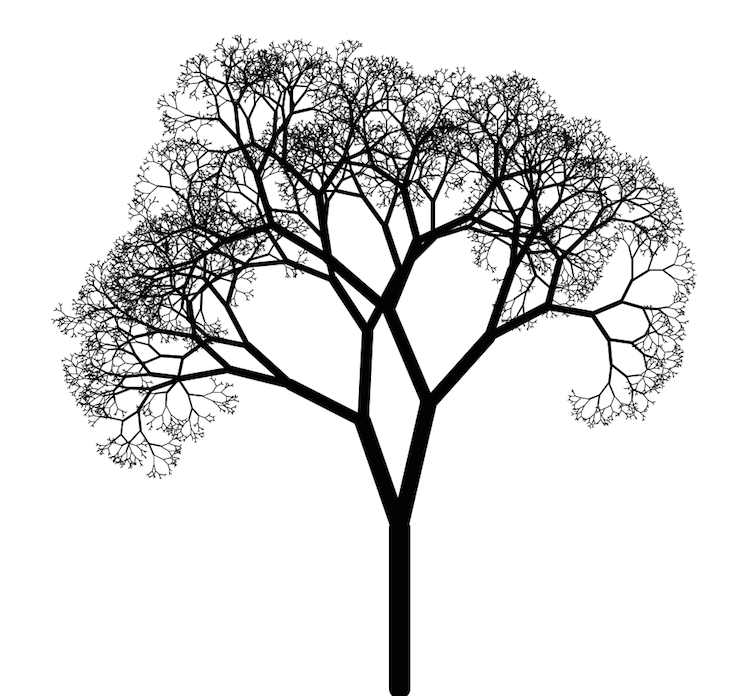Take a screenshot of your tree drawing and upload it as part of your HW4 submission using the submit script.

### [10 points] The Sierpinski Triangle

Next we'll draw another fun fractal shape called the Sierpinski triangle. Here is what it looks like taken out to six generations: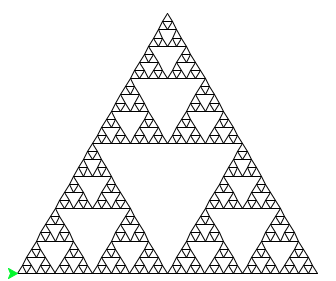There are many ways to draw this shape, but we are going to use the same technique that we used for the Koch curve. Rather than taking a line and breaking it into thirds, we are going to draw half size triangles. Here is an abstraction of how Generation N of the Sierpinski Triangle is built: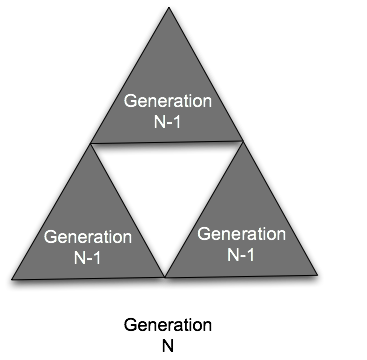More simply, here are the first generations 1, 2, 3, 4: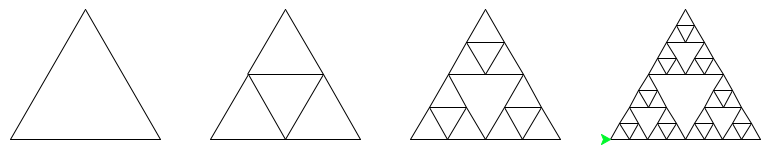Here is one of the easiest ways to draw the Sierpinski Triangle. Start with the assumption that when you draw the triangle, the turtle will be left in the same position and orientation it started from. Given that, drawing a generation N triangle just involves drawing three N-1 triangles that are half the size. Draw the shape, move to the location of the next one, draw again, move to the last triangle and draw that one, then restore the turtle to the original location and orientation. For generation 0, don’t do anything (think of the base case of the spiral rather than the Koch curve).

All of this should go in a function called ```drawSierpinski(length, iterations)```. `length` will be the length of the base of the figure and iterations will tell us how many generations to make. You should also include the following function to call your function and set everything up:

``````def drawSierpinskiScene(length, iterations):
"""
Use this code to call your drawSierpinski() function. This provides
the functionality of turning off the tracer and running turtle.done().
"""

# turn off animation (too slow otherwise)
turtle.tracer(False)

# pick up the pen and move the turtle so it starts at the left edge of the canvas
turtle.penup()
turtle.goto(-turtle.window_width()/3 + 20,0)
turtle.pendown()

drawSierpinski(length, iterations)

# finish
turtle.update()
turtle.done()

``````

Take a screenshot of your Sierpinski Triangle for generation 4 and upload it as part of your HW4 submission using the submit script.

### [2 extra points] Optional Challenge

Write a new recursive function (and a helper function that sets up the turtle and calls your function) to draw the Sierpinski carpet. You may find it useful to pick up and put down the pen while doing the drawing. Feel free to enhance your carpet with color! Here are generations 0 through 4: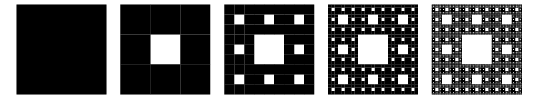### Turtle reference

Here are the most important commands from the Python `turtle` library (more can be found in the online documentation).

`forward(distance)`
Move the turtle forward by the specified `distance` in the direction it is facing.
`backward(distance)`
Move the turtle backwards by the specified `distance` in the opposite direction to where it is facing.
`right(angle)`
Turn the turtle to its right by the specified `angle`. Note that this can be any angle, not the four cardinal directions we had with LightBot.
`left(angle)`
Turn the turtle to its left by the specified `angle`.
`goto(x, y)`
Drive the turtle immediately to the specified location without changing its orientation.
`pendown()`
Put the pen down so that the movement of the turtle leaves a track.
`penup()`
Pick the pen up so that there are no tracks when the turtle moves.
`width(linewidth)`
Set the thickness of the line left behind by the turtle.
`pencolor(colorstring)`
Change the color of the pen that the turtle is using. This can be a string such as ‘red’, ‘yellow’, ‘blue’, etc. Of course, you have to pick a valid color name.
`fillcolor(colorstring)`
When this is set, the turtle will try to “fill in” any closed shapes that you draw with the given color.
`begin_fill()`
Call this before starting to draw a filled shape.
`end_fill()`
Call this function when you have finished drawing a shape that you want to be filled. This tells the turtle the shape is done and that it can try and fill it in.
`tracer(state)`
Turn on or off watching the turtle trace out the shapes. This is useful when you are trying to draw complex shapes and don’t want to wait. Pass in either `True` or `False` to turn the tracing on or off.
`update()`
If you turn off tracing, you should can use this function to get everything the turtle has drawn so far to appear.
`window_width()`
Returns the current width of the turtle window.
`window_height()`
Returns the current height of the current turtle window.